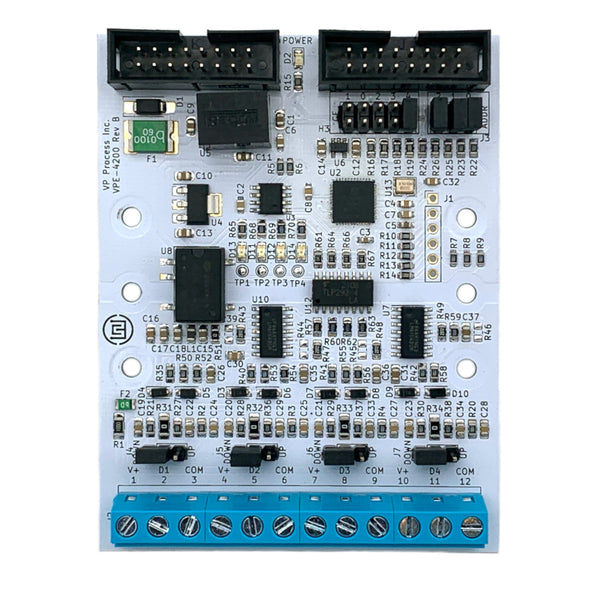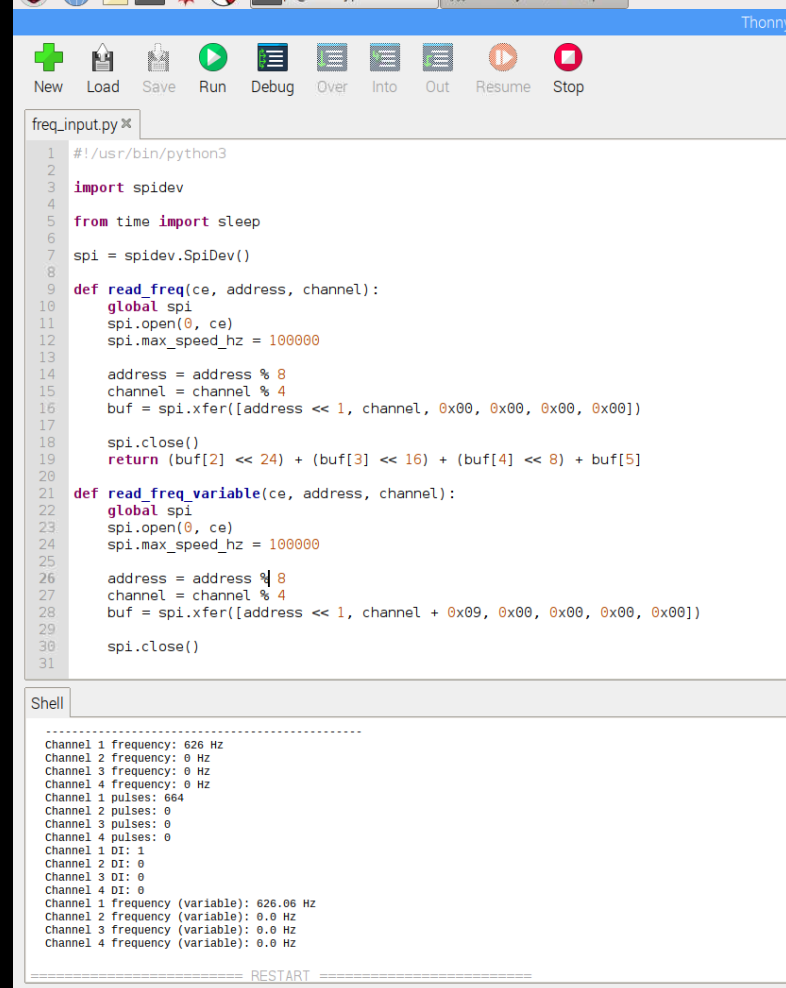# PI-SPI-DIN-4FREQ Frequency / Pulse Counter

Regular price \$125.00 USD Sale

The PI-SPI-DIN-4FREQ is a four channel isolated Frequency /  Pulse Counter / Digital Input module for the PI-SPI-DIN series. The SPI communication allows for simple register reads of input values.

### SPECIFICATIONS:

• All Functions run Simultaneously allowing any combination of inputs types
• Frequency measurement with fixed (one second) or variable (up to 100 second) sample period
• Five jumper selectable CE (Chip Enable) lines
• Three Address Jumpers allows for 8 units per CE selection
• 0-24 VDC OR 0-15 VAC capable inputs
• NPN, PNP or FLOATING input per channel (Jumper Selectable)
• Optical Isolation between inputs to SPI communication and power
(Inputs are not isolated from each other)
• Frequency Range: 0.01 Hz to 100 kHz
• Resolution: 0.001 Hz
• Trigger Voltage: 2.5V (Min) under ideal conditions
(Can be adjusted by simple resistor value change)
• DC coupling (Default)
• AC coupling (Requires removal of shorting resistor)
• Input Amplification capability (Requires installation of Gain resistor on a channel by channel basisThe PI-SPI-DIN-4FREq is available in two versions:

PI-SPI-DIN-4FREQ-PCB as a pcb module

PI-SPI-DIN-4FREQ-DIN complete with DIN rail mounting clips

### Default limits for Frequency Counter:

2.5V min at 0 - 5 KHz sine wave
2.6V min at 5 - 15 KHz sine wave
2.7V min at 15 - 25 KHz sine wave
2.8V min at 25 - 35 KHz sine wave
2.9V min at 35 - 50 KHz sine wave
3.1V min at 50 - 55 KHz sine wave
3.2V min at 55 - 60 KHz sine wave
3.3V min at 60 - 65 KHz sine wave
3.4V min at 65 - 70 KHz sine wave
3.6V min at 70 - 75 KHz sine wave
3.7V min at 75 - 80 KHz sine wave
3.9V min at 80 - 85 KHz sine wave
4.0V min at 85 - 90 KHz sine wave
4.1V min at 90 - 95 KHz sine wave
4.2V min at 95 - 100 KHz sine wave

2.5V min at 5 - 35 KHz square wave
2.6V min at 35 - 60 KHz square wave
2.7V min at 60 - 75 KHz square wave
2.8V min at 75 - 85 KHz square wave
2.9V min at 85 - 100 KHz square wave

### Sample Python Code:

``` #!/usr/bin/python3import spidevfrom time import sleepspi = spidev.SpiDev()def read_freq(ce, address, channel):    global spi    spi.open(0, ce)    spi.max_speed_hz = 100000        address = address % 8    channel = channel % 4    buf = spi.xfer([address << 1, channel, 0x00, 0x00, 0x00, 0x00])        spi.close()    return (buf << 24) + (buf << 16) + (buf << 8) + bufdef read_freq_variable(ce, address, channel):    global spi    spi.open(0, ce)    spi.max_speed_hz = 100000        address = address % 8    channel = channel % 4    buf = spi.xfer([address << 1, channel + 0x09, 0x00, 0x00, 0x00, 0x00])        spi.close()        cycles = (buf << 24) + (buf << 16) + (buf << 8) + buf    value = round(24000000 / cycles, 3) if cycles > 0 else 0.0        return valuedef read_pulse(ce, address, channel):    global spi    spi.open(0, ce)    spi.max_speed_hz = 100000        address = address % 8    channel = channel % 4    buf = spi.xfer([address << 1, channel + 0x04, 0x00, 0x00, 0x00, 0x00])        spi.close()    return (buf << 24) + (buf << 16) + (buf << 8) + bufdef read_di(ce, address, channel):    global spi    spi.open(0, ce)    spi.max_speed_hz = 100000        address = address % 8    channel = channel % 4    buf = spi.xfer([address << 1, 0x08, 0x00, 0x00, 0x00, 0x00])        spi.close()    return (buf >> channel) & 1while True:    ce = 0 # CE0-4 jumpers    address = 0 # ADDR 0-2 jumpers        # Read frequency counter    print('\n------------------------------------------------')    print('Channel 1 frequency:', read_freq(ce, address, 0), 'Hz')    print('Channel 2 frequency:', read_freq(ce, address, 1), 'Hz')    print('Channel 3 frequency:', read_freq(ce, address, 2), 'Hz')    print('Channel 4 frequency:', read_freq(ce, address, 3), 'Hz')        # Read pulse counter    print('Channel 1 pulses:', read_pulse(ce, address, 0))    print('Channel 2 pulses:', read_pulse(ce, address, 1))    print('Channel 3 pulses:', read_pulse(ce, address, 2))    print('Channel 4 pulses:', read_pulse(ce, address, 3))        # read DI    print('Channel 1 DI:', read_di(ce, address, 0))    print('Channel 2 DI:', read_di(ce, address, 1))    print('Channel 3 DI:', read_di(ce, address, 2))    print('Channel 4 DI:', read_di(ce, address, 3))        print('Channel 1 frequency (variable):', read_freq_variable(ce, address, 0), 'Hz')    print('Channel 2 frequency (variable):', read_freq_variable(ce, address, 1), 'Hz')    print('Channel 3 frequency (variable):', read_freq_variable(ce, address, 2), 'Hz')    print('Channel 4 frequency (variable):', read_freq_variable(ce, address, 3), 'Hz')        sleep(1) ```

### Sample Screen Shot: